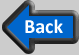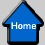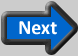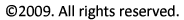Work, Heat, and Energy

To evaluate a propulsion system or propulsion device , it is necessary to see it in action. From the point of view of thrust, the action we are interested in is motion of the object to which thrust is applied. We call the effect of a force on a moving object the work done by the force. The work done (WT) by a constant thrust force (FT) on an object that moved a distance ΔL in the direction of the thrust is calculated as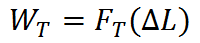If this work is done over a time period, Δt, the rate at which work is done (called power) is given by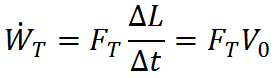where V0 = ΔL/Δt is the object velocity. Since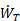is the rate of work done by thrust, it is often called thrust power.Example Problem: Cycling

The capacity to do work is provided by energy. In particular, energy is required to generate the thrust force that moves an airplane through the sky or a fish through the ocean. Energy comes in many different forms, several of which are described below.

• Kinetic energy is energy related to object motion. An object of mass m moving at a speed V has kinetic energy ½mV2. An object moving at a high velocity has a lot of kinetic energy and therefore, a large capacity to do work. For example, it takes a high speed car a longer distance to slow to a stop because the brakes must do more work to stop the vehicle. Similarly, a high velocity bat can hit a baseball much further.• Potential energy most commonly refers to gravitational potential energy, which is energy associated with an object’s elevation. For an object of mass m and elevation z, its potential energy is mgz where g is the gravitational constant (9.81 m/s2). Potential energy may be thought of as “stored” kinetic energy. For instance, an object dropped from a high elevation can reach a large velocity (large kinetic energy) as potential energy is converted to kinetic energy during the fall.

•• Internal energy is energy related to the motion of molecules comprising an object. A high temperature object has a lot of internal energy (its molecules are moving vigorously) and vice versa.In the container on the left, the gas molecules are hot and moving very rapidly, whereas in the container on the right, the gas is cold and the molecules are moving slowly. The high temperature gas contains more internal energy (per unit mass) than the cold gas. For gases in enclosed containers, a higher temperature also leads to increased pressure, as represented by the larger mass holding the piston in place for the container on the left.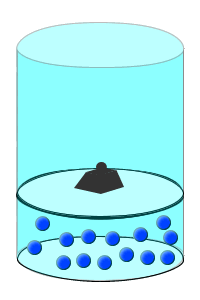• Chemical energy is energy stored in chemical bonds. Hydrocarbon fuels like octane (the main component in automobile gasoline) contain a lot of chemical energy, which can be released through combustion (burning) and used by internal combustion engines to do work.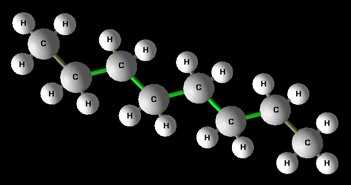A related quantity not to be confused with energy is heat. Heat is energy in transit between two objects of different temperature. That is, energy transfer between two objects at different temperatures occurs by heat. This process is also referred to as heat transfer. For example, a frozen steak in a hot pan will tend to heat up while the pan tends to cool down due to heat transfer by conduction from the pan to the steak. Heat transfer may also occur by convection and radiation, but the detailed physics behind heat transfer are beyond the scope of this discussion. The main point is to recognize that energy can enter and leave an object by heat.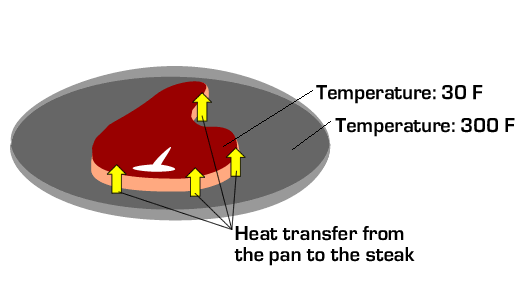1st Law of Thermodynamics:

There is a special relationship between work, energy, and heat described by the First Law of Thermodynamics. In words, the first law states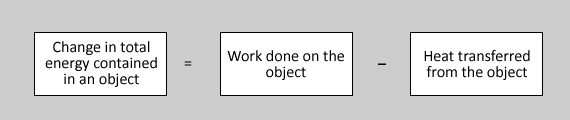or mathematically,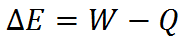Note that energy can be contained by an object (it is a property of an object), but work and heat cannot. Rather, work and heat are actions done by an object (an object can do work and it can lose energy by heat, but it does not contain work or heat).Example Problem: Jet Fuel Energy

The 1st Law of Thermodynamics allows us to analyze the interaction between thrust work and the energy that provided the capacity to do the work. In particular, a key observation derived from the 1st Law of Thermodynamics is that energy is required to do work. Either the internal energy stores of an object must be depleted (ΔE < 0) or energy must be transferred to the object by heat (Q < 0) for it to do work (W < 0). This provides the basis for evaluating the efficiency of a propulsion system.